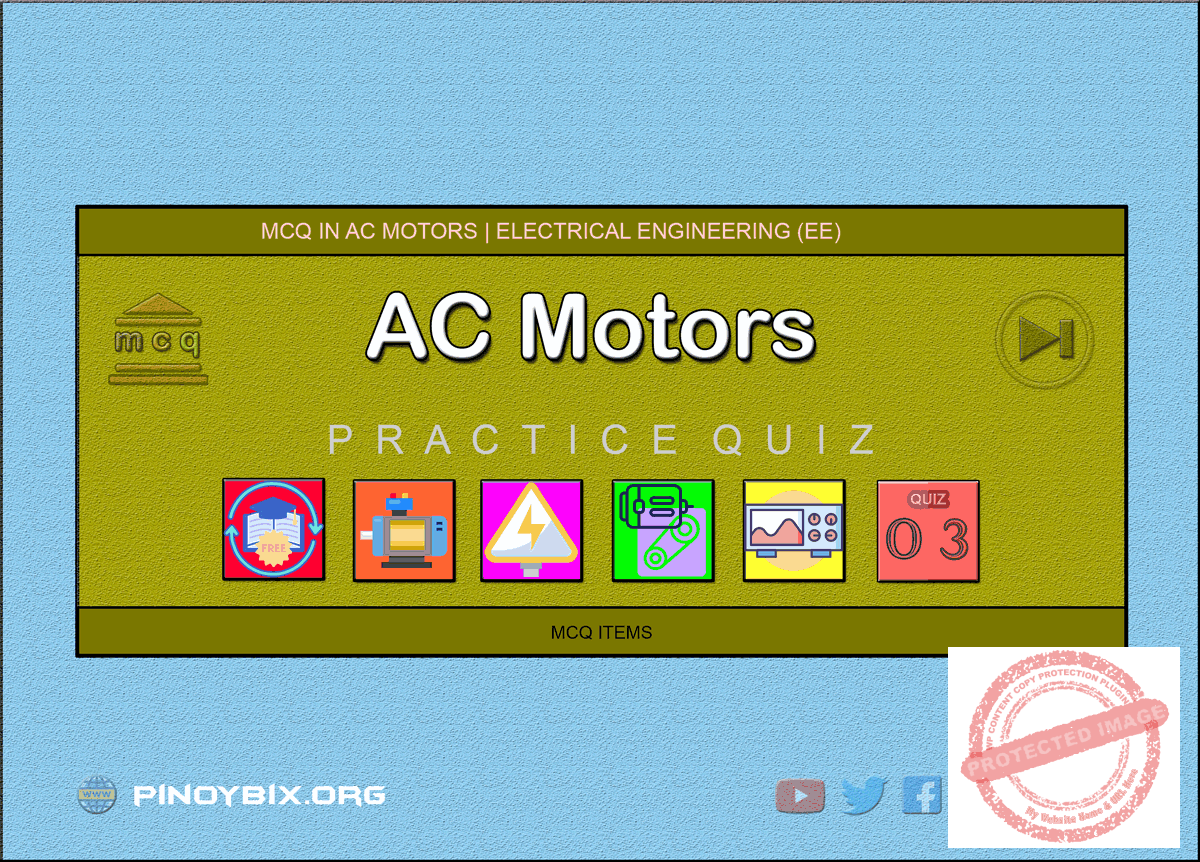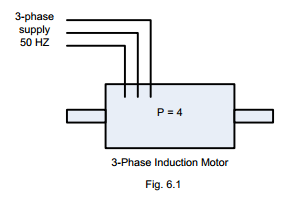# MCQ in AC Motors Part 3 | REE Board Exam

(Last Updated On: August 8, 2020)This is the Multiple Choice Questions Part 3 of the Series in AC Motors as one of the Electrical Engineering topic. In Preparation for the REE Board Exam make sure to expose yourself and familiarize in each and every questions compiled here taken from various sources including but not limited to past Board Exam Questions in Electrical Engineering field, Electrical Engineering Books, Journals and other Electrical Engineering References.

#### Continue Practice Exam Test Questions Part 3 of the Series

Choose the letter of the best answer in each questions.

101.  When a 3-phase induction motor is at no load, the slip is

A.  1

B.  0.5

C.  practically zero

D.  0.2

Solution:

102.  When the rotor of a 3-phase induction motor is blocked, the slip is

A.  zero

B.  0.5

C.  0.1

D.  1

Solution:

103.  The speed of the rotating flux in Fig. 6.1 will beA.  1500 rpm

B.  1000 rpm

C.  750 rpm

D.  none of these

Solution:

104.  If the induction motor shown in Fig. 6.1 runs at 1450 rpm, then slip is

A.  50%

B.  3.3%

C.  5%

D.  none of these

Solution:

105.  In Fig. 6.1, the rotor frequency ____ is when the motor is at standstill.

A.  zero

B.  25 Hz

C.  50 Hz

D.  none of these

Solution:

106.  If one of the lines in Fig. 6.1 is interchanged, then

A.  flux speed increases

B.  flux speed decreases

C.   flux speed remains the same but direction is reversed

D.  none of these

Solution:

107.  If a 4-pole induction motor has a synchronous speed of 1500 rpm, then supply frequency is

A.  50 Hz

B.  25 Hz

C.  60 Hz

D.  none of these

Solution:

108.  The full-load slip of a 3-phase induction motor ranges from

A.  10% to 20%

B.  20% to 30%

C.  2% to 5%

D.  none of these

Solution:

109.  The direction of rotation of field in a 3-phase induction motor depends upon

A.  number of poles

B.  magnitude of supply voltage

C.  supply frequency

D.  phase sequence of supply voltage

Solution:

110.  The rotor winding of a 3-phase wound rotor induction motor is generally ____ connected.

A.  star

B.  delta

C.  partly star partly delta

D.  none of these

Solution:

111.  3-phase wound rotor motors are also called ____ motors.

A.  synchronous

B.  slip ring

C.  series

D.  commutator

Solution:

112.  The advantage of wound rotor motor is that

A.  it is inexpensive

B.  it requires less maintenance

C.  external resistance can be inserted in the rotor circuit

D.  none of these

Solution:

113.  A wound rotor motor is mainly used in application where

A.  a high starting torque is required

B.  speed control is not required

C.  less costly motor is required

D.  high rotor resistance is required during running

Solution:

114.  The torque characteristic of a 3-phase induction motor is similar to that of

A.  dc series motor

B.  dc shunt motor

C.  dc differentially compounded motor

D.  dc cumulatively compounded motor

Solution:

115.  In a wound rotor motor, the rotor winding is wound for poles ____ that of the stator winding.

A.  equal to

B.  greater than

C.  smaller than

D.  none of these

Solution:

116.  Wound rotors are less extensively used than squirrel cage motor because

A.  slip rings are required on the rotor circuit

B.  rotor windings are generally Y-connected

C.  they are costly are required greater maintenance

D.  none of these

Solution:

117.  A 4-pole, 50 Hz induction motor operates at 5% slip. The frequency of emf induced in the rotor will be

A.  25 Hz

B.  50 Hz

C.  2.5 Hz

D.  none of these

Solution:

118.  The maximum voltage is induced in the rotor of a 3-phase induction when it

C.  is blocked

D.  none of these

Solution:

119.  The reactance of the rotor circuit of a 3-phase induction motor is maximum at

D.  starting

Solution:

120.  The rotor current in a 3-phase induction motor is ____ slip.

A.  inversely proportional to

B.  directly proportional to

C.  independent of

D.  none of these

Solution:

121.  At starting, rotor reactance of a 3-phase induction motor is ____ rotor resistance.

A.  small as compared to

B.  equal to that of

C.  large as compared to

D.  none of these

Solution:

122.  When an induction motor is running at full-load, rotor reactance is ____ rotor resistance.

A.  comparable to

B.  very large compared to

C.  large compared to

D.  none of these

Solution:

123.  If the slip of a 3-phase induction motor increases, the p.f. of the rotor circuit

A.  is increased

B.  is decreased

C.  remains unchanged

D.  none of these

Solution:

124.  The magnetizing current drawn by a 3-phase induction motor is about ____ of full-load stator current.

A.  5%

B.  10 to 15%

C.  15 to 20%

D.  30 to 50%

Solution:

125.  A high starting torque can be obtained in a 3-phase induction motor by

A.  increasing rotor resistance

B.  decreasing rotor resistance

C.  increasing rotor reactance

D.  none of these

Solution:

126.  The starting torque of a 3-phase induction motor is ____ supply.

A.  independent of

B.  directly proportional to

C.  directly proportional to the square of

D.  none of these

Solution:

127.  The starting torque of an induction motor is maximum when rotor resistance per phase is ____ rotor reactance/phase.

A.  equal to

B.  two times

C.  four times

D.  none of these

Solution:

128.  Under running conditions, the maximum torque of 3-phase induction motor will occur at that value of slip at which rotor resistance/phase is ____ rotor reactance/phase.

A.  two times

B.  four times

C.  equal to

D.  none of these

Solution:

129.  The maximum torque of a 3-phase induction motor under running conditions is

A.  inversely proportional to supply voltage

B.  inversely proportional to rotor reactance at standstill

C.  directly proportional to rotor resistance

D.  none of these

Solution:

130.  If the supply voltage of a 3-phase induction motor is increased two times, then torque is

A.  increased two times

B.  decreased two times

C.  increased four times

D.  decreased four times

Solution:

131.  The 3-phase induction motor is so designed that the rotor should have ____ under running conditions.

A.  high resistance

B.  high reactance

C.  large slip

D.  low resistance

Solution:

132.  If a 3-phase induction motor is running at slip s (in decimal) then rotor copper loss is equal to

A.  (1 – s) x rotor input

B.  (1 + s) x rotor input

C.  s x rotor input

D.  none of these

Solution:

133.  If an induction motor is running at slip s (in decimal) then rotor output is equal to

A.  (1 + s) x rotor input

B.  (s – 1) x rotor input

C.  s x rotor input

D.  (1 – s) x rotor input

Solution:

134.  If Ns and Nr are the speeds of rotating field and rotor respectively, the ratio rotor input/rotor output is equal to

A.  Nr/Ns

B.  Ns/Nr

C.  Ns – Nr

D.  Nr – Ns

Solution:

135.  At no-load, the rotor core loss of a 3-phase induction motor is

A.  large

B.  small

C.  practically small

D.  none of these

Solution:

136.  Friction and windage loss of a 3-phase induction motor are

C.  remains the same at all loads

D.  none of these

Solution:

137.  For higher efficiency of 3-phase induction motor, the slip should be

A.  large

B.  very large

C.  as small as possible

D.  1

Solution:

138.  If a 3-phase induction motor is running at a slip s (in decimal), then approximate efficiency of the motor is

A.  s2

C.  s – 1

B.  1 + s

D.  1 – s

Solution:

139.  A 3-phase induction motor is running at 2% slip. If the input to rotor is 1000 W, then mechanical power developed by the motor is

A.  20 W

B.  980 W

C.  500 W

D.  200 W

Solution:

140.  The approximate efficiency of a 3-phase 50 Hz, 4-pole induction motor running at 1350 rpm is

A.  90%

B.  40%

C.  65%

D.  none of these

Solution:

141.  In a squirrel cage rotor, the bars are not placed to the shaft but are skewed to have

A.  greater mechanical strength

B.  less rotor losses

C.  uniform torque

D.  none of these

Solution:

142.  In a squirrel cage motor, the number of stator slots is ____ rotor slots.

A.  always equal to the number of

B.  always greater than the number of

C.  always less than the number of

D.  either more or less than the number of

Solution:

143.  If the air gap between the rotor and stator of 3-phase induction motor is increased then

B.  leakage reactances are decreased

C.  leakage reactances are increased

D.  none of these

Solution:

144.  If the slip of induction motor increases, then current in the stator winding

A.  is increased

B.  is decreased

C.  remains unchanged

D.  none of these

Solution:

145.  The conditions of an induction motor at no-load resemble those of a transformer whose secondary is

A.  short-circuited

B.  open-circuited

C.  supplying a variable resistive load

D.  none of these

Solution:

146.  The conditions of an induction motor at standstill resemble those of a transformer whose secondary is

A.  short-circuited

B.  open-circuited

C.  supplying a variable resistive load

D.  none of these

Solution:

147.  The conditions of an induction motor on load resemble those of a transformer whose secondary is

A.  short-circuited

B.  open-circuited

C.  supplying a variable resistive load

D.  none of these

Solution:

148.  The speed of a squirrel cage induction motor is changed by

A.  pole changing

B.  rheostatic control

D.  none of these

Solution:

149.  One of the speeds of a 2-speed squirrel cage induction motor is 800 rpm (lower speed). The other speed would be

A.  400 rpm

B.  2400 rpm

C.  1600 rpm

D.  1200 rpm

Solution:

150.  A four speed squirrel cage induction motor uses ____ stator windings.

A.  four

B.  three

C.  one

D.  two

Solution:

P inoyBIX educates thousands of reviewers and students a day in preparation for their board examinations. Also provides professionals with materials for their lectures and practice exams. Help me go forward with the same spirit.

“Will you subscribe today via YOUTUBE?”

Subscribe

PinoyBIX Engineering. © 2014-2020 All Rights Reserved | How to Donate? |